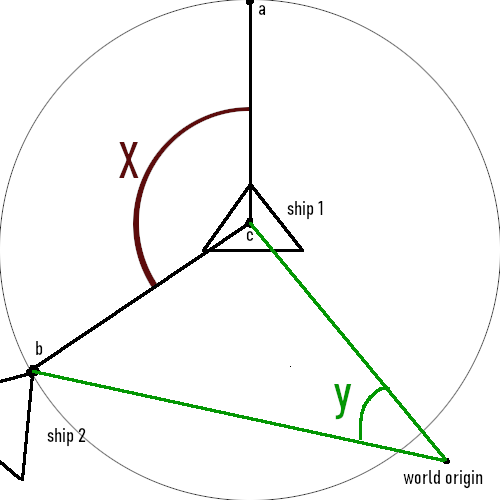# trying to measure the angle between 3 points

So i have a 2d space game. I have a ship, call it ship 1. it has a circle collider around it. Another ship, ship 2, touches the collider. I want to measure the angle between where ship 1 is facing and where ship 2 touched the collider.

I’ve stumbled across Vector2.Angle and Vector2.SignedAngle but neither of these seem helpful as they use the world origin point 0,0,0 as the vertex. When i looked around online there are a lot of guides and posts about “finding the angle between two objects” but the thing is that you cant measure an angle with only 2 points, you need 3. For this reason I find it a bit confusing when the unity api claims that this function “Gets the signed angle in degrees between from and to.” the angle between two points is just 0.

If i spawn ship 2 on the same exact y coordinates as ship 1 and the ships are facing each other, then when i fly into the collider i should get an angle of 0. but using those functions i get an angle of about -3.1. And no matter where around the collider i collide, i never get an angle more than about 3.9, and its because its actually measuring the diameter of the collider, from the origin.

most people online seem to advise using these same methods though, and they often include the same line of debugging code that draws a line between the two points, but this line is deceiving. at first i saw the line and got some numbers and thought it was working but the numbers are wrong. I feel like either I must be missing something or everyone else is. I dont see much value in measuring the angle between two objects from the origin personally, how often do you actually do that? surely you should be able to specify the vertex point.

I’ve made a figure that shows two different angles, x and y. i believe angle y is the angle that angle and signedangle are measuring but what i actually want is angle x.

note i also tried using the Vector3 versions of these methods as well but depending on which axis you cancel i either get the same numbers or i get all zeros from every angle.for clarity heres the code i have currently. like i said the numbers are all wrong, whats weird is they sometimes flip from negative to positive seemingly randomly.

``````private void OnTriggerEnter2D(Collider2D collision)
{
if (collision.gameObject.CompareTag("spaceship"))
{
Vector2 a = this.transform.position;
Vector2 b = collision.transform.position;
float angle = Vector2.SignedAngle(a, b);
Debug.DrawLine(a, b, Color.white, 2.5f, false);
Debug.Log("Angle is " + angle + " degrees.");

if (angle > 0)
{
steeringInput = -1;
}
if (angle < 0)
{
steeringInput = 1;
}
}
}
``````

@mmajcan

so first off, collision.transform.position; is not the contact point of the colision, and thus can be off. you get it with collision.GetContacts or something like that, but you only if you use OnCollision (on Trigger has not that data).
Anyway, When you take than one Vector3 p = contact.point (or collision.transform.position), you just calculate Vector3 toPoint = (p - this.transform.position).nomalize; and then with Mathf.acos(Vector3.dot(toPoint, this.transform.forward))
you calculate the angle between the two ships. The angle is relative though, but that is what you described with angle X

As @GamerLordMat says you need to take the direction from the ship to the point of impact and then calculate the angle between that and the ship’s forward vector. I think it can be done with signed angle.

``````Vector2 Other = collision.transform.position;
Vector2 directionToOther = other - transform.position;

float angle = Vector2.SignedAngle(transform.forward, directionToOther);
``````

Side note: This assumes that when your ship is not rotated it’s front points to the right.